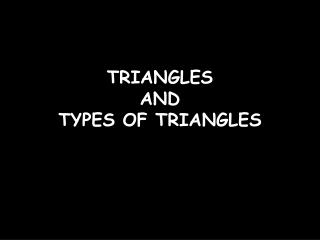DownloadDownload PresentationTRIANGLES AND TYPES OF TRIANGLES

# TRIANGLES AND TYPES OF TRIANGLES

Download Presentation## TRIANGLES AND TYPES OF TRIANGLES

- - - - - - - - - - - - - - - - - - - - - - - - - - - E N D - - - - - - - - - - - - - - - - - - - - - - - - - - -
##### Presentation Transcript

1. TRIANGLES AND TYPES OF TRIANGLES

2. We get a TRIANGLE by joining three non collinear points .

3. What is a TRIANGLE ? • A closed figure formed by joining three line segments is called a TRIANGLE .

4. A triangle has three sides

5. A triangle has three angles

6. TYPES OF TRIANGLES Two Ways • On the basis of angles • On the basis of sides

7. On the basis of ANGLES Three types • Acute angled triangle • Right angled triangle • Obtuse angled triangle

8. Acute angled triangle • A triangle whose all the angles are acute is called an ACUTE ANGLED TRIANGLE .

9. Right angled triangle • A triangle in which one of the angle is right angle is called RIGHT ANGLED TRIANGLE.

10. Obtused angled triangle • A triangle in which one of the angle is obtuse angle is called OBTUSED ANGLED TRIANGLE .

11. On the basis of sides Three types • Equilateral triangle • Isosceles triangle • Scalene triangle

12. Equilateral triangle • A triangle whose all the sides are of equal length is called equilateral triangle.

13. Isosceles triangle • A triangle whose any two sides are of equal measure is called scalene triangle.

14. Scalene triangle • A triangle whose no two sides are of equal measure is called scalene triangle.

15. Recap • A closed figure formed by joining three non collinear points is called • A triangle whose all the sides are of equal length is called • A triangle whose no two sides are of equal length is called • A triangle whose one angle is 90 degree is called • A triangle whose all the angles are less than 90 degree is called